### IMO Shortlist 1994 problem A2

Kvaliteta:
Avg: 0,0
Težina:
Avg: 6,0
Let$m$ and$n$ be two positive integers. Let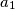$a_1$,$a_2$,$\ldots$,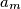$a_m$ be$m$ different numbers from the set$\{1, 2,\ldots, n\}$ such that for any two indices$i$ and$j$ with$1\leq i \leq j \leq m$ and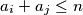$a_i + a_j \leq n$, there exists an index$k$ such that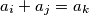$a_i + a_j = a_k$. Show that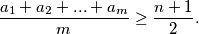Izvor: Međunarodna matematička olimpijada, shortlist 1994